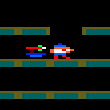### CPC Basic

Would you like to react to this message? Create an account in a few clicks or log in to continue.

# Moving Pixels Down the ScreenOut Bush

Posts : 11
Join date : 2019-12-02
Like my Vertical Stars earlier, this deals with the problem of when Pixels reach the bottom of the screen, so numbers need to be able to divide into 2 and when 2 is reached move back to the top and a new random position along the x coordinate assigned.

Code:
`100 DEFWORD a-z:DIM a(30)110 FOR n=1 TO 30 STEP 3120  x=n+1130  y=n+2140  a(x)=RND MOD 640150  o=RND MOD 398160  WHILE o MOD 2 <> 0170    o=RND MOD 398180  WEND190  a(y)=o200 NEXT n210 MODE 0:INK 0,0:INK 1,26220 WHILE 1230  FOR n=1 TO 30 STEP 3240  x=n+1:y=n+2250  IF a(y)>2 THEN PLOT a(x),a(y),0:a(y)=a(y)-2:PLOT a(x),a(y),1260  IF a(y)=2 THEN PLOT a(x),a(y),0:GOSUB 290:PLOT a(x),a(y),1270  NEXT n280 WEND290 a(x)=RND MOD 640300 a(y)=398310 RETURN`

Current date/time is Thu Aug 18, 2022 6:55 pm